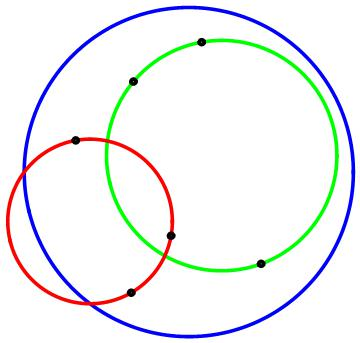# Gianlino's Disc

Calculus Level 5

Three points are randomly chosen from the interior of an unit circle.The probability that the circumcircle passing through those three points lies entirely inside the unit circle can be expressed as $\dfrac { A }{ B }$, where $A$ and $B$ are co-prime integers.

Find $\displaystyle { A }^{ 2 }+{ B }^{ 2 }.$

This problem is not original, credit goes to "Gianlino". A proof of this would be nice.

×

Problem Loading...

Note Loading...

Set Loading...